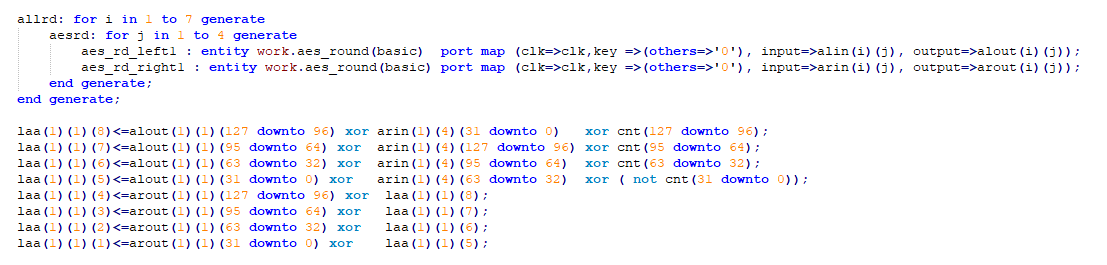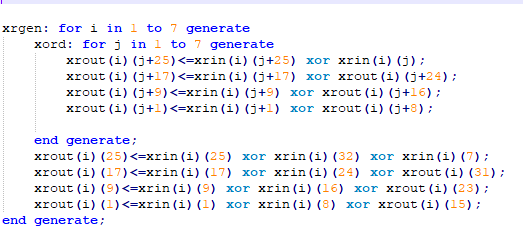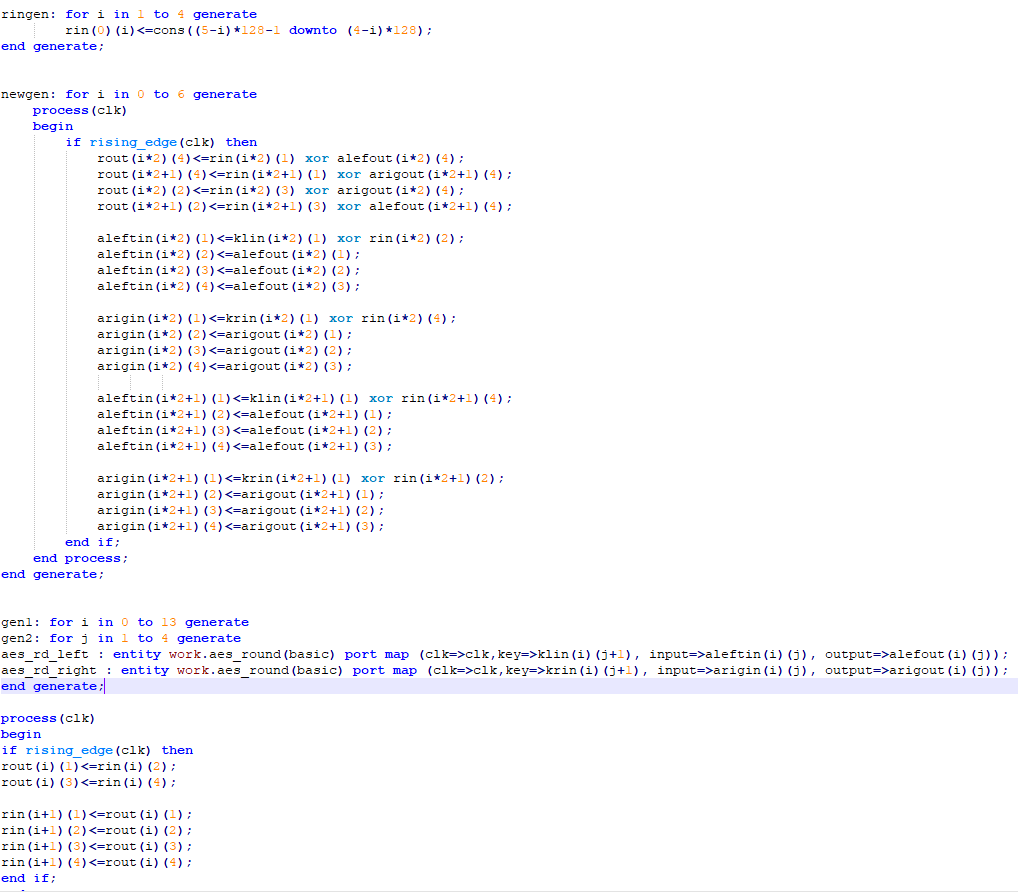# Shavite-3 Reference Guide

#### Shavite-3 Reference Guide for Crypto-algorithms [RG-S3]

Fully pipelined and efficient implementation of Shavite-3 with 600Mh/s hash rate per core

##### PREFACE:

This is FPGA implementation of Shavite-3 hash function with 512-bit digest. This is meant to be used for mining purpose. Hence the input message size is assumed to be 512 – 640 bit. The target device used for analysis VCU1525 .

##### METHODOLOGY:

To make the length of message consistent for the round, the message is padded to make it multiple of 1024.  The steps of padding are:

• Append bit 1 after the end of input message.
• Add zeros until the total length of message is 1024*n +880 (where n={0,1,2,3,…..}.
• Add the length of original message in 128-bit format.
• Add the length of digest in 16-bit format.

The total length after padding should be integer multiple of 1024. For our purpose with 512-640 bit message length, the total size of the message is just 1024-bit.

###### Message Expansion:

The message expansion accepts 1024-bit block,128-bit counter & 512-bit salt which are treated as arrays of 32-bit words. So, block hash 32 words, counter 4 words and salt have 16 words. They are used to generate 112 subkeys of 128-bit each totaling 448 32-bit words. For our convenience we can basically ignore the salt because it is just zero.

Let rk [.] be 448 32-bit array, msg [0,….,31] be message array, cnt [0,..,3] be counter and salt [0,….,15] be salt array. Then the first 32 words of rk is initialized by our input message themselves. Then, we repeat a process that generates 32 words in a nonlinear manner and 32 words in a linear manner. Sixteen of the produced words are XORed with the counter (four with each cnt[i], where four times the XORed counter word is complemented.

We use linear method and non-linear method alternatively.

###### Non-linear expansion:

For fully pipelined implementation, we put 8 AES round all with zero key. Input to all these AES rounds is given by the last expanded message (previous key). First key is the message input itself. The output of these AES rounds is scrambled and xored with the cnt to get the new key. This key is used for the rounds and also for next key generation with linear method.

Here is small segment of the non-linear expansion. Out of 14 rounds of message expansion 7 of them are done non-linear way. In each, there are 8 AES round as we can see in the code below. We derive alin(AES left in) ,arin(AES right in) from the previous key or msg itself. Laa here is the level after Aes which we get from xoring AES in/out with cnt. The laa values are the required keys for next expansion.###### Linear expansion:

For linear expansion , the key is basically xored internally with itself. The input to this is from the output of non-linear expansion and its output also goes to non-linear expansion. Here xrin is the input to linear expansion and xrout is the output.###### Round

There are 14 rounds in shavite-3 tweaked and updated version. The input to these rounds is 512-bit value and keys which are generated from message expansion. The input to first round is constant. Each round consists of pair of four AES rounds (left and right) totaling 8 AES rounds.

Let klin(0,1,2,3) and krin(0,1,2,3) be the expanded key of message left and right respectively.

Let , left Aes input and output be alefin and alefout. Similarly with right AES.

Then we can get round ouput as follows.###### Changes from the Tweaked/updated Submission

We can notice from the code above that in alternating rounds the input to AES rounds are changed. This difference improves the security without influencing the performance.

##### CONCLUSION:

This is fully pipelined design which is meant to be implemented at very high frequency in FPGA. So, we kept no more than three xors between two flip-flops. The dominant consumption of this design was 125783 or 10.64% LUT in VCU1525.